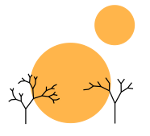# Transfinite Induction

Rimu Shuang. "Untitled Photo". Jan 23, 2014. Under a Creative Commons 3.0 Attribution License.

### math

NOT FINISHED: Just to comply with Beeminder’s deadlines, getting something out

Before continuing with the constructible universe and V = L, it is first necessary to take a look rigorously at what transfinite induction is. I won’t prove a lot of what I say here, just lay the formal groundwork so that we can understand why V = L is in fact a first-order axiom. Most of the time, it is sufficient to think of transfinite induction as ordinary induction plus the limit ordinal case. In other words, if we provide a base case, a successor ordinal case, and a limit ordinal case, then we’ve shown that something is true for all ordinals. To put this more rigorously, we’ll start with defining well-founded relations.

I will use < instead of the more common R to describe the relations we’ll talk about here because although we not have the property of transitivity, in most other ways, the relations we’re talking about act very much like orders. A well-founded relation < on a class C is a linear relation such that every non-empty set S which is a subset of C has a <-minimal element where a <-minimal element in a set S is an element e such that x ∈ S(e < x) and moreover that the class ext<(x){yCy<x} is a set (i.e. that < is set-like). We have to define one last term which is pred<(x) = {yXyTC(<)x} where TC is the transitive closure of <.

Then transfinite induction put rigorously refers to the theorem that if < is a well-founded relation on a class C and X ⊆ C such that x ∈ X(pred<(x) ⊂ X → x ∈ X), then X = C. In this case we’ll say that two classes C1 and C2 are equal under a well-founded relation < if for all x in C1, x is in C2 and the sets Si = {yCiy<x} are equal and vice versa (switching C2 for C1). To see that this accords with the informal definition provided at the beginning of this post, let C be the class of all ordinals. Then what the theorem states is that if we have a statement such that its truth for all ordinals less than some ordinal τ implies its truth for τ, then the statement is true for all ordinals. Hence this ends up in practice reducing to just checking that something holds for the next successor ordinal and then checking that it holds for the next limit ordinal because every ordinal is either a successor ordinal or a limit ordinal.

Transfinite induction yields transfinite recursion, a method of constructing mathematical objects beyond normal recursion. The formal statement is that given a well-founded relation < on a class C and a function G : C × X → X, there is a unique function F : C → X such that c ∈ C(F(c) = G(c, F ↾ pred<(c))) where F ↾ S = {(x,y)∈FxS}. To see again how this ends up working in practice, let’s begin with a slightly less general definition and work through how the Von Neumann universe V = ∪α ∈ OrdVα is constructed.

1. V0 = ∅

2. Vα + 1 = 𝒫(Vα)

3. Vλ = ∪α < λVα if λ is a limit ordinal

Our slightly less general definition of transfinite recursion is the following: Given an ordinal α, for all β < α let gβ be a function β → Ord, let f be a function Ord → Ord and let G : B → Ord where B = {gββ<α}. Then there is a unique F : Ord → Ord such that F(0) = α0, F(β + 1) = f(F(β)) if β + 1 ∈ α and F(λ) = G(F ↾ λ) if λ ∈ α.

In the case of the Von Neumann universe, to see how this plays out, let’s fix some limit ordinal τ. Then for all β < τ let gβ : β ↦ β and